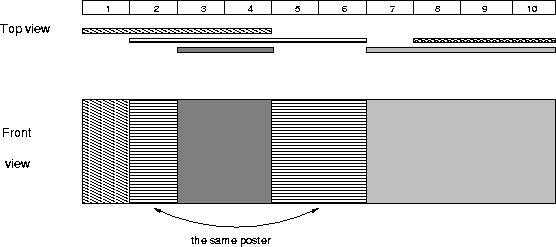## poj 2528

2019年02月28日 算法 ⁄ 共 3435字 ⁄ 字号 评论关闭
Mayor's posters
 Time Limit: 1000MS Memory Limit: 65536K Total Submissions: 33753 Accepted: 9788

Description

The citizens of Bytetown, AB, could not stand that the candidates in the mayoral election campaign have been placing their electoral posters at all places at their whim. The city council has finally decided to build an electoral wall for placing the posters
and introduce the following rules:
• Every candidate can place exactly one poster on the wall.
• All posters are of the same height equal to the height of the wall; the width of a poster can be any integer number of bytes (byte is the unit of length in Bytetown).
• The wall is divided into segments and the width of each segment is one byte.
• Each poster must completely cover a contiguous number of wall segments.

They have built a wall 10000000 bytes long (such that there is enough place for all candidates). When the electoral campaign was restarted, the candidates were placing their posters on the wall and their posters differed widely in width. Moreover, the candidates
started placing their posters on wall segments already occupied by other posters. Everyone in Bytetown was curious whose posters will be visible (entirely or in part) on the last day before elections.
Your task is to find the number of visible posters when all the posters are placed given the information about posters' size, their place and order of placement on the electoral wall.

Input

The first line of input contains a number c giving the number of cases that follow. The first line of data for a single case contains number 1 <= n <= 10000. The subsequent n lines describe the posters in the order in which they were placed. The i-th line among
the n lines contains two integer numbers li and ri which are the number of the wall segment occupied by the left end and the right end of the i-th poster, respectively. We know that for each 1 <= i <= n, 1 <= li <= ri <= 10000000. After
the i-th poster is placed, it entirely covers all wall segments numbered li, li+1 ,... , ri.

Output

For each input data set print the number of visible posters after all the posters are placed.

The picture below illustrates the case of the sample input.Sample Input

```1
5
1 4
2 6
8 10
3 4
7 10
```

Sample Output

```4
```

Source

1
3
1 10
1 3
6 10

```#include<iostream>
#include<cstdio>
#include<cstring>
#include<algorithm>
#include<map>
using namespace std;
struct node
{
int l,r;
int v;
}t;
int c,n,a,b,d,num,ans;
bool vis;
int p;
void pushdown(int rt)
{
if(t[rt].v)
{
t[rt<<1].v=t[rt].v;
t[rt<<1|1].v=t[rt].v;
t[rt].v=0;
}
}
void create(int ll,int rr,int rt)
{
if(ll>rr)return;
t[rt].l=ll;
t[rt].r=rr;
t[rt].v=0;
if(ll==rr)return;
int m=(ll+rr)/2;
create(ll,m,rt<<1);
create(m+1,rr,rt<<1|1);
}
void update(int ll,int rr,int rt,int va)
{
if(t[rt].l>t[rt].r||ll>rr)return;
if(t[rt].l==ll&&t[rt].r==rr)
{
t[rt].v=va;
return;
}
else
{
if(t[rt].v)pushdown(rt);
int m=(t[rt].l+t[rt].r)/2;
if(rr<=m)update(ll,rr,rt<<1,va);
else if(ll>m)update(ll,rr,rt<<1|1,va);
else
{
update(ll,m,rt<<1,va);
update(m+1,rr,rt<<1|1,va);
}
}
}
void query(int rt)
{
if(t[rt].l>t[rt].r)return;
if(t[rt].v)
{
if(vis[t[rt].v]==0)
{
ans++;
vis[t[rt].v]=1;
}
}
else
{
if(t[rt].l==t[rt].r)return;
query(rt<<1);
query(rt<<1|1);
}
}
int main()
{
scanf("%d",&c);
while(c--)
{
scanf("%d",&n);
for(int i=0;i<n;i++)
{
scanf("%d%d",&a[i],&b[i]);
d[i<<1]=a[i];
d[i<<1|1]=b[i];
}
sort(d,d+(n<<1));
memset(p,0,sizeof(p));
num=0;
int nn=(n<<1);
for(int i=0;i<nn;i++)
{
if(p[d[i]]==0)p[d[i]]=++num;
}
for(int i=0;i<n;i++)
{
a[i]=p[a[i]];
b[i]=p[b[i]];
}
create(1,num,1);
for(int i=0;i<n;i++)
{
update(a[i],b[i],1,i+1);
}
memset(vis,0,sizeof(vis));
ans=0;
query(1);
cout<<ans<<endl;
}
return 0;
}
```
【上篇】
【下篇】